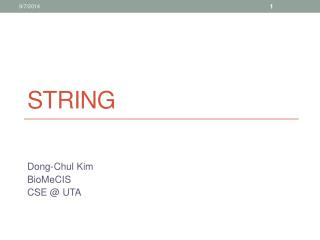DownloadDownload PresentationString

# String

Télécharger la présentation## String

- - - - - - - - - - - - - - - - - - - - - - - - - - - E N D - - - - - - - - - - - - - - - - - - - - - - - - - - -
##### Presentation Transcript

1. String Dong-Chul Kim BioMeCIS CSE @ UTA

2. 1D Array and 2D Array • When declaring a 2D array, the number of columns must be stated. • Example • int array1D[] = {1, 2, 3}; • int array2D[] = { {4, 5, 6},{7, 8, 9} };

3. The char Data Type • A char is a one byte integer type typically used for storing characters. • Example: • char oneLetter = ’D’; • We enclose the character in single quotes, not double quotes.

4. ASCII • Plain text is often stored in a format called ASCII (American Standard Code for Information Interchange). There are 128 characters in ASCII, which includes the standard alphanumeric characters as well as some non-printable characters (e.g., tab, newline, and so forth).

5. char Example • A char variable can be initialized using the character or the decimal value of the character. • #include <stdio.h> • int main(void) • { • char letter_a = ’a’; /* note the use of single quotes */ • char decimal_a = 97; /* 97 is the decimal value • for ’a’ in ASCII */ • /* note the %c to print characters */ • printf("letter_a is %d and %c\n", letter_a, letter_a); • printf("decimal_a is %d and %c\n", decimal_a, decimal_a); • } • Output • letter_a is 97 and a • decimal_a is 97 and a

6. char cont. • If we check an ASCII chart, we see that the letters A–Z have ASCII values of 65–90 while a–z have values of 97–122. Because the letters are stored as numbers, we can perform numeric operations on them. • #include <stdio.h> • int main(void) • { • char uppercase = ’A’; /* 65 in ASCII */ • char lowercase; • lowercase = uppercase + 32; • printf("Adding 32 to %c gives us %c\n", uppercase, lowercase); • } • prints • Adding 32 to A gives us a

7. String • A character string is a series of one or more characters. • Example • “I am at UTA” • The double quotation marks are not part of the string. They just inform the compiler they enclose a string, just as single quotation marks identify a character.

8. Array of char • C does not have a string type. Characters in a string are stored in adjacent memory cells, one character per cell, and an array consists of adjacent memory locations, so placing a string in an array is quite natural. • We use an array of chars for storing a string. • We can declare this just like we did with other types. • Example • char letters = “I am at UTA”;

9. What if the input string has a length more than the size of the array ? • Is there any application to use this point? • \0 is the null character which is used by C for marking the end of a string. • The null character is not the digit zero; it is the nonprinting character. • Its ASCII code value is 0. • The presence of the null character means the array must have at least one more cell than the number of the characters to be stored • char letters[as long as > 11] = “I am at UTA”;

10. Even though we define a char array much larger than a string, the null character will tell the compiler where is the end of the string. This is useful when we call printf function.

11. Scanf a string and print it out • #include <stdio.h> • int main(void) • { • char name;// you can’t use name[], the size has to be fixed. • printf("What's your name?\n"); • scanf("%s", name); • printf("Hello, %s.\n", name); • return 0; • } • %s is the format specifier for a string. • The name of a char array represents an address in computer memory, therefore, we don’t need “&” when we call scanf function.

12. Access characters in a string • #include <stdio.h> • int main(void) • { • char text[] = "I am at UTA"; • int i = 0; • i = 0; • while(text[i] != '\0') /*use '\0' as terminal*/ • { • printf("%c %3d\n", text[i], text[i]); • i++; • } • }

13. String length • #include <stdio.h> • #include <string.h> • int main(void) • { • char text; • int size, i; • printf("Enter a string: "); • scanf("%s", text); • printf("you entered %s\n", text); • size = strlen( text ); • printf("The number of characters in the input string is %d.\n", size); • return 0; • } • The strlen function returns the number of characters in a string, excluding ‘\0’. • strlen(the name of a char array) • Call strlen function needs “#include <string.h>”

14. Note • If your input is • ABCD edEc asdetE • Just calling scanf() once will only store ABCD in the char array as a string. The remaining of the input will be ignored. The space is used to separate strings in a sentence.

15. Now if we access all characters of a string in a loop, we have two approaches to terminate the loop: • (1) the length of the string ---- strlen() • (2) ‘\0’

16. Reverse a string • #include <stdio.h> • #include <string.h> • int main(void) • { • char text; • int size, i; • printf("Enter a string: "); • scanf("%s", text); • printf("you entered %s\n", text); • size = strlen( text ); • for(i = size - 1; i >= 0; i--) • printf("%c", text[i]); • printf("\n"); • }